Cart0 items
Total price \$0

# Technical support

### Harmonic distortion

Harmonic distortion of the main power source is a switching phenomenon, especially the high speed power switches found in Variable Frequency Drives.

22.12.2021

### Calculating the Position

The receiver identifies every satellite by a comparison of the received codes with those stored in the receiver. The receiver can then calculate the positions of the satellites in space using the orbital data that have been transmitted in the navigational signal.

The receiver measures the distance to a satellite by the time required for the signal to travel from the satellite to the receiver (time-of-flight).

This is done by the receiver generating an exact copy of the code transmitted by the satellite, which are then compared by a so-called correlation technique. This measures the lag between the signals and is converted into the distance to the satellite. Measurement errors can arise and include:

• Errors in the satellite clock
• Errors in the receiver clock
• Errors caused by delays in the signal on its passage through the ionosphere and the troposphere.
The receiver compensates for errors in the satellite clock and for ionospheric distortion using a mathematical model to generate a "pseudorange'7. The difference between the pseudorange and the true distance depends on errors in the receiver clock and any remaining ionospheric effects. Cost considerations dictate that the receiver clock has not the same quality as the atomic clocks in the satellites.
The effect of the receiver clock errors is that the position spheres don't cross each other at a point. The clock error affects all the measurements to the same extent,so the receiver performs a series of iterative calculations to adjust the position circles until they finally intersect one another at a point. This result gives the receiver clock error and is used in the calculations that follow.
The receiver has to make at least four time measurements in order to determine its own clock error and to give one position in space. Five measurements give a validation of the position. Even more measurements gives a greater margin of safety to I errors and a more accurate position. The distance to the receiver is calculated in the same right-angular x,y,z coordinate system used for the satellites' positions. The receiver then converts the calculated position to latitude and longitude using the WSG-84 geoi as used by GPS.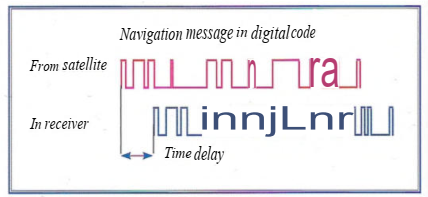Two distances in space give two position spheres which intersect in a circle. A third measurement gives two positions on thatcircle,afourth distancegives a single position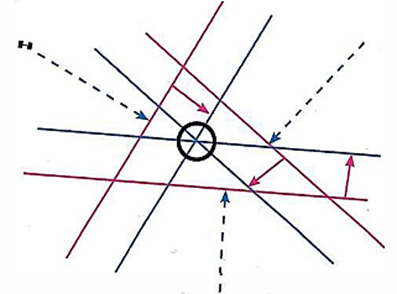Position lines on the surface of the earth. A mathematical method reiterates all the pseudoranges position lines to a single position, as a!! the ranges contain the same error (red components). For simplicity this diagram is constructed in two dimensions although the satellite positions are three-dimensional1.  Navigational information

The central function of the navigational system is to calculate the position of the vessel, which can then be plotted on the chart. Because the system also has memory, processor and clock, it can also generate other useful parameters such as COG, SOG, older positions (to show the Past Track) and a COGvector based on these older positions.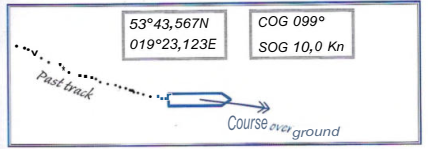Speed ever Ground (SOG) and Course over Ground (COG) are calculated averages over a user-defined time interval

A Waypoint (WP) can be entered to mark a position for course alteration. Entering a series of Waypoint Positions will create a route. Then the GNSS receiver can calculate: distance, trip distance, ETA, Cross Track Error (XTE), Cross Track Distance (XTD).
The GNSS-set can only get the bearing and distance to the next Waypoint if the route has been activated.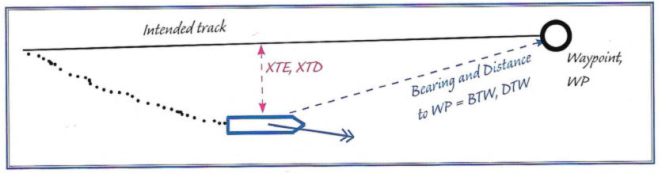Normally the GNSS will present the position on an electronic chart, which requires the use of a computer or plotter. When the connection to an electronic chart has been established then further functions can be introduced, such as an alarm for the risk of grounding.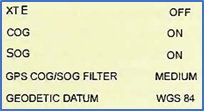1.1. Settings

The GPS satellite navigation system uses a description of the shape of the Earth which is called WGS 84 (World Geodetic System 84, or just WGS). Among the many settings on the navigation system is one for the geodetic system and it is important that this setting corresponds to the geodetic system for the chart or electronic chart being used.

There are more than 100 geodetic systems with advantages and disadvantages for various places on the globe. To get the most out of the navigational system, the Navigator has to enter, or at any rate check, a number of settings so that he is in control of what is shown and knows how it is calculated.

Back to the list